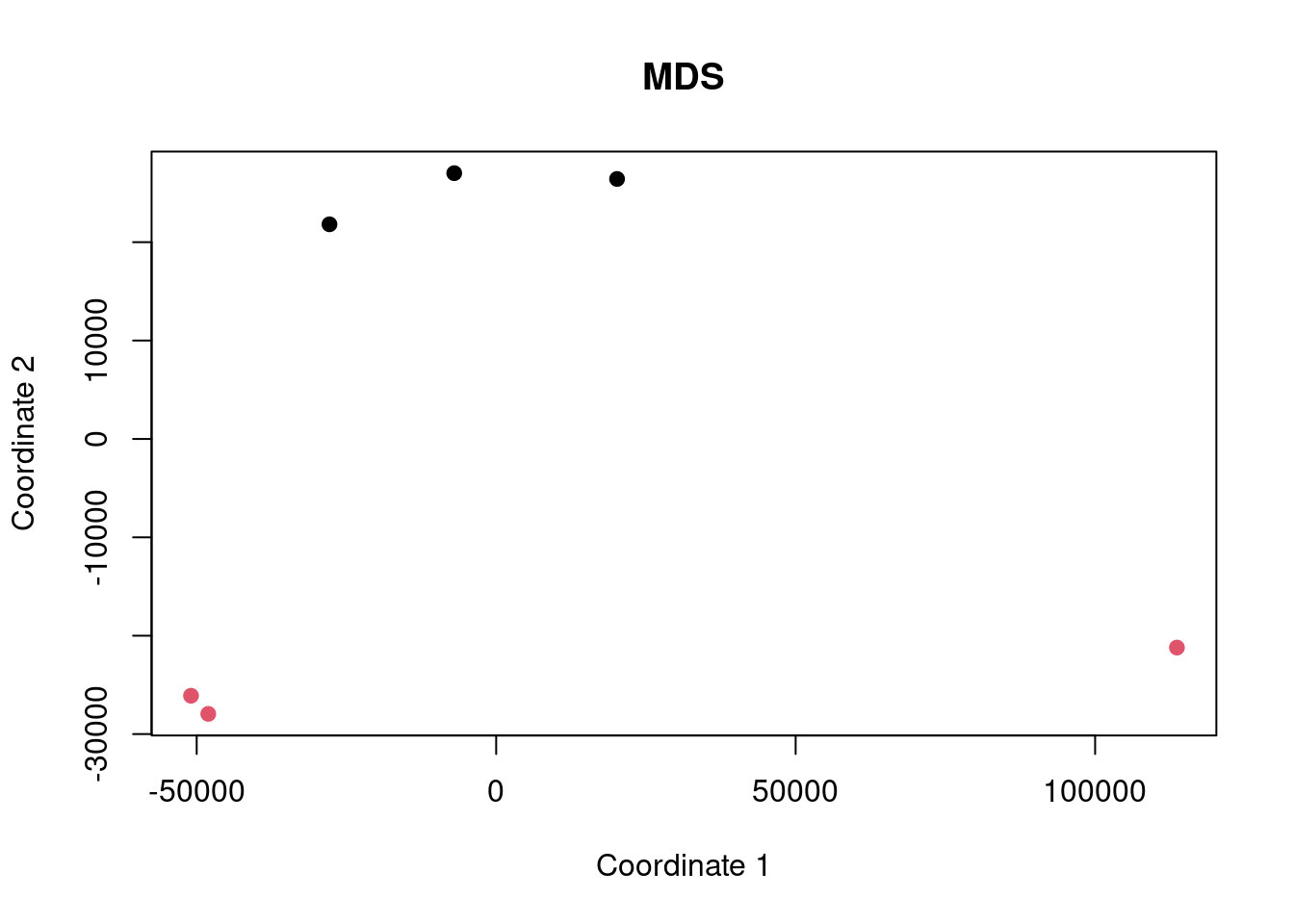## Intro

Here we are performing an analysis of some gene expression data to demonstrate the difference between ORA and FCS methods and to highlight the differences caused by improper background gene set use.

The dataset being used is SRP038101 and we are comparing the cells grown in normal condition (control) to those grown with addition of Azacitidine (case).

Data are obtained from http://dee2.io/

``````suppressPackageStartupMessages({
library("getDEE2")
library("DESeq2")
library("clusterProfiler")
library("mitch")
library("kableExtra")
library("eulerr")
})``````

## Get expression data

I’m using some RNA-seq data looking at the effect of Azacytidine on AML3 cells.

``````name="SRP038101"
samplesheet <- mdat[grep("SRP038101",mdat\$SRP_accession),]
samplesheet<-samplesheet[order(samplesheet\$SRR_accession),]
samplesheet\$trt<-as.factor(c(1,1,1,0,0,0))
s1 <- samplesheet

s1 %>% kbl(caption = "sample sheet") %>% kable_paper("hover", full_width = F)``````
sample sheet
SRR_accession QC_summary SRX_accession SRS_accession SRP_accession Sample_name GEO_series Library_name trt
239157 SRR1171523 PASS SRX472607 SRS559064 SRP038101 GSM1329859 GSE55123 1
239158 SRR1171524 WARN(3,4) SRX472608 SRS559066 SRP038101 GSM1329860 GSE55123 1
239159 SRR1171525 WARN(3,4) SRX472609 SRS559065 SRP038101 GSM1329861 GSE55123 1
239160 SRR1171526 WARN(3,4) SRX472610 SRS559068 SRP038101 GSM1329862 GSE55123 0
239161 SRR1171527 WARN(3,4) SRX472611 SRS559067 SRP038101 GSM1329863 GSE55123 0
239162 SRR1171528 WARN(3,4) SRX472612 SRS559069 SRP038101 GSM1329864 GSE55123 0
``w<-getDEE2("hsapiens",samplesheet\$SRR_accession,metadata=mdat,legacy = TRUE)``
``````## For more information about DEE2 QC metrics, visit
##     https://github.com/markziemann/dee2/blob/master/qc/qc_metrics.md``````
``````x<-Tx2Gene(w)
x<-x\$Tx2Gene

# save the genetable for later
gt<-w\$GeneInfo[,1,drop=FALSE]
gt\$accession<-rownames(gt)

# counts
x1<-x[,which(colnames(x) %in% samplesheet\$SRR_accession)]``````

Here show the number of genes in the annotation set, and those detected above the detection threshold.

``````# filter out lowly expressed genes
x1<-x1[which(rowSums(x1)/ncol(x1)>=(10)),]
nrow(x)``````
``##  39297``
``nrow(x1)``
``##  13926``

Now multidimensional scaling (MDS) plot to show the correlation between the datasets. If the control and case datasets are clustered separately, then it is likely that there will be many differentially expressed genes with FDR<0.05.

``plot(cmdscale(dist(t(x1))), xlab="Coordinate 1", ylab="Coordinate 2", pch=19, col=s1\$trt, main="MDS")``## Differential expression

Now run DESeq2 for control vs case.

``y <- DESeqDataSetFromMatrix(countData = round(x1), colData = s1, design = ~ trt)``
``## converting counts to integer mode``
``y <- DESeq(y)``
``## estimating size factors``
``## estimating dispersions``
``## gene-wise dispersion estimates``
``## mean-dispersion relationship``
``## final dispersion estimates``
``## fitting model and testing``
``````de <- results(y)
de<-as.data.frame(de[order(de\$pvalue),])
rownames(de)<-sapply(strsplit(rownames(de),"\\."),"[[",1)
head(de) %>% kbl() %>% kable_paper("hover", full_width = F)``````
baseMean log2FoldChange lfcSE stat pvalue padj
ENSG00000090382 14491.5013 1.686516 0.0460876 36.59368 0 0
ENSG00000165949 1288.2858 3.326521 0.1053480 31.57651 0 0
ENSG00000275214 911.4085 3.432709 0.1151500 29.81075 0 0
ENSG00000115461 615.6738 5.004631 0.1746428 28.65638 0 0
ENSG00000111331 2366.8131 2.649803 0.0945138 28.03615 0 0
ENSG00000157601 1153.6028 2.820804 0.1016830 27.74116 0 0

Now let’s have a look at some of the charts showing differential expression. In particular, an MA plot and volcano plot.

``````maplot <- function(de,contrast_name) {
sig <-subset(de, padj < 0.05 )
up <-rownames(subset(de, padj < 0.05 & log2FoldChange > 0))
dn <-rownames(subset(de, padj < 0.05 & log2FoldChange < 0))
GENESUP <- length(up)
GENESDN <- length(dn)
DET=nrow(de)
SUBHEADER = paste(GENESUP, "up, ", GENESDN, "down", DET, "detected")
ns <-subset(de, padj > 0.05 )
plot(log2(de\$baseMean),de\$log2FoldChange,
xlab="log2 basemean", ylab="log2 foldchange",
pch=19, cex=0.5, col="dark gray",
main=contrast_name, cex.main=0.7)
points(log2(sig\$baseMean),sig\$log2FoldChange,
pch=19, cex=0.5, col="red")
}

make_volcano <- function(de,name) {